# Value iteration networks

Value Iteration Networks Tamar et al., NIPS 2016

‘Value Iteration Networks’ won a best paper award at NIPS 2016. It tackles two of the hot issues in reinforcement learning at the moment: incorporating longer range planning into the learned strategies, and improving transfer learning from one problem to another. It’s two for the price of one, as both of these challenges are addressed by an architecture that learns to plan.

In the grid-world domain shown below, a standard reinforcement learning network, trained on several instances of the world, may still have trouble generalizing to a new unseen domain (right-hand image).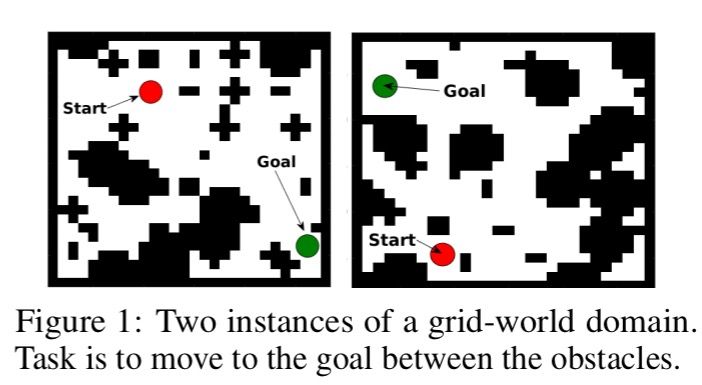(This setup is very similar to the maze replanning challenge in ‘Strategic attentive writer for learning macro actions‘ from the Google DeepMind team that we looked at earlier this year. Both papers were published at the same time).

… as we show in our experiments, while standard CNN-based networks can be easily trained to solve a set of such maps, they do not generalize well to new tasks outside this set, because they do not understand the goal-directed nature of the behavior. This observation suggests that the computation learned by reactive policies is different from planning, which is required to solve a new task.

Planning is not a new problem – the value iteration algorithm based on Markov decision processes (MDP) has been known since 1957! What Tamar et al. do in this work though, is embed a value iteration (VI) planning component inside the overall neural network architecture. And the breakthrough insight is that the VI algorithm itself can be encoded by a specific type of CNN, which means it is differentiable.

By embedding such a VI network module inside a standard feed-forward classification network, we obtain an NN model that can learn the parameters of a planning computation that yields useful predictions. The VI block is differentiable, and the whole network can be trained using standard backpropagation.

It really is pretty cool – you give the network the machinery that can be used for planning, and it figures out all by itself the best way to use it.

Using the approach, Tamar et al. show that value iteration networks (VINS) generalize better to new grid-world scenarios than either CNNs following the DQN architecture, or fully convolutional networks (FCNs):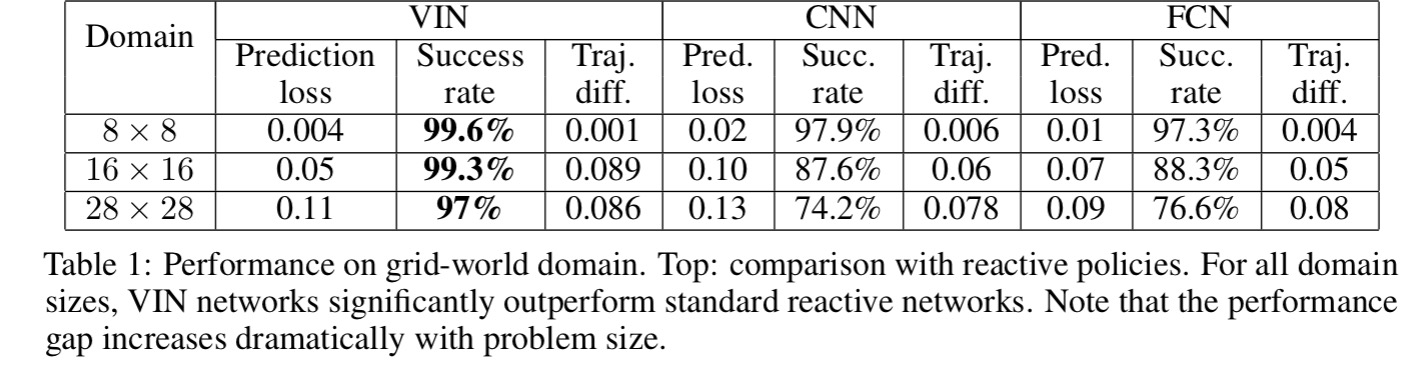(Note there is no comparison to the contemporary STRAW architecture from the DeepMind team that also extends DQNs with planning).

Importantly, note that the prediction loss for the reactive policies is comparable to the VINs, although their success rate is significantly worse. This shows that this is not a standard case of overfitting/underfitting of the reactive policies. Rather, VIN policies, by their VI structure, focus prediction errors on less important parts of the trajectory, while reactive policies do not make this distinction, and learn the easily predictable parts of the trajectory yet fail on the complete task.

They also demonstrated planning success using Mars landscape images for Mars Rover navigation, planning in a physical simulation setting, and planning in the WebNav setting which requires navigating links of a web site towards a goal page.

What I’d love to see is how well the VIN architecture performs on the Frostbite Challenge.

Let’s take a closer look at how it all works, starting with the value iteration algorithm itself, then how to encode that in a NN, before finally putting it all together in a complete architecture.

### Standard value iteration

“A standard model for sequential decision making and planning is the Markov Decision Process (MDP).”

You have a set of states$s \in S$, a set of actions$a \in A$, a reward function$R(s,a)$ that gives the anticipated reward for taking action$a$ in state$s$, and a transition kernel,$P(s'|s,a)$ that encodes the probability of the next state given the current state and action. A policy$\pi(a|s)$ prescribes the action distribution for each state.

(Note the similarity between this structure and the action matrix of STRAW).

The goal in an MDP is to find a policy that obtains high rewards in the long term.

You can consider the value of a state under some policy as the expected discounted sum of rewards when starting from that state and following the policy. A optimal policy will find the maximal long-term return possible from a given state. Value iteration computes the rewards by iterating over the action steps ($\gamma \in (0,1)$ is a discount factor):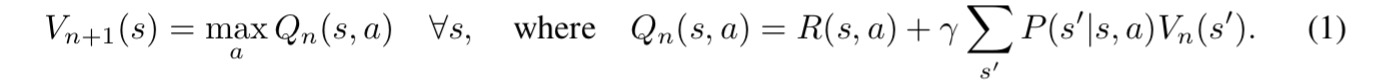### Encoding value iteration in a neural network

Our starting point is the VI algorithm (1). Our main observation is that each iteration of VI may be seen as passing the previous value function Vn and reward function R through a convolution layer and max-pooling layer. In this analogy, each channel in the convolution layer corresponds to the Q-function for a specific action, and convolution kernel weights correspond to the discounted transition probabilities. Thus by recurrently applying a convolution layer K times, K iterations of VI are effectively performed.

This idea leads to the following network structure: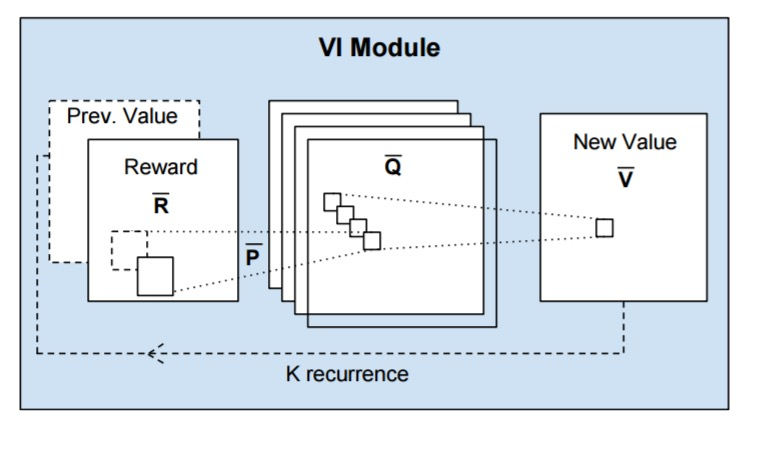A reward ‘image’$\bar{R}$ (to follow the more normal CNN formulation of working with images) is fed into convolutional layer$\bar{Q}$ with$\bar{A}$ channels. Each channel corresponds to$\bar{Q}(\bar{s},\bar{a})$ for action$\bar{a}$. The layer is max-pooled along the actions channel to produce the next-iteration value function layer. This is stacked with the reward$\bar{R}$ and fed back in K times, to perform K iterations of value iteration.

### The full Value Iteration Network model

The value-iteration module we just described can now be embedded into a full value iteration network as follows: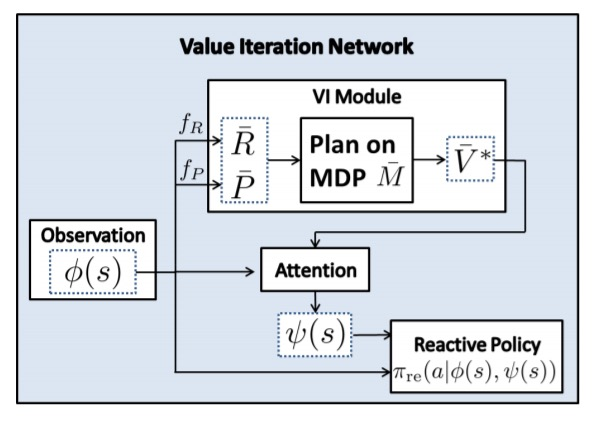In many systems, if you’re in a given state, and you take a given action, the set of possible states you end up in is much smaller than the overall universe of states. More precisely, the the states for which$\bar{P}(\bar{s'}|\bar{s},\bar{a}) > 0$ is a small subset of$\bar{S}$.

In NN terminology, this is a form of attention, in the sense that for a given label prediction (action), only a subset of the input features (value function) is relevant. Attention is known to improve learning performance by reducing the effective number of network parameters during learning.

This is the purpose of the attention module added into the feedback loop in the diagram above. With the inclusion of the CNN-based value iteration module, everything in the value iteration network is differentiable:

This allows us to treat the planning module as just another NN, and by back-propagating through it, we can train the whole policy end-to-end.

To implement a VIN, you need to specify the state and action spaces for the planning module ($\bar{S}$ and$\bar{A}$), the reward functions$f_R$ and$f_P$, and the attention function. The authors call this the process of VIN design.

Once a VIN design is chose, implementing the VIN is straightforward, as it is simply a form of CNN. The networks in our experiments all required only several lines of Theano code.